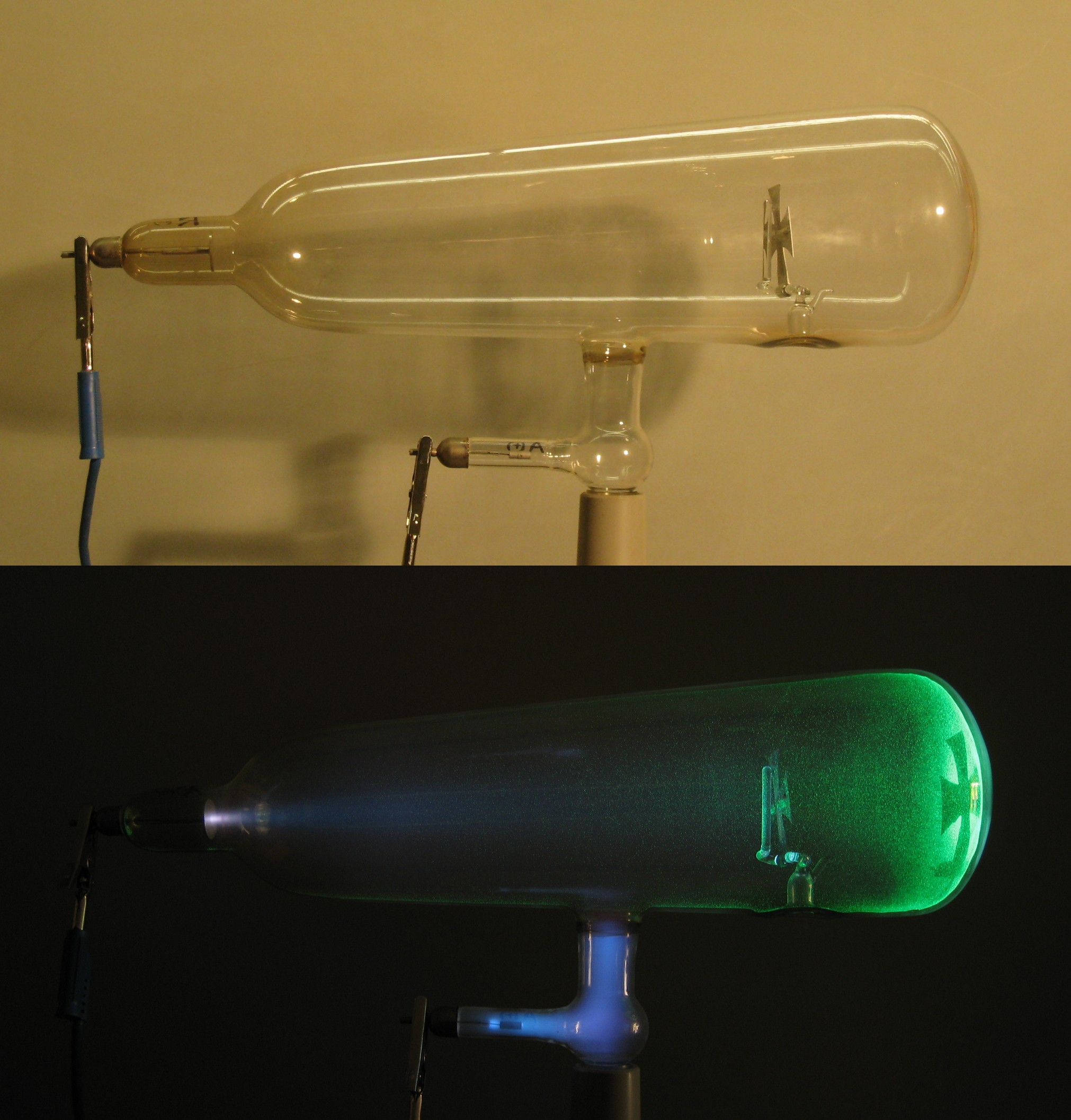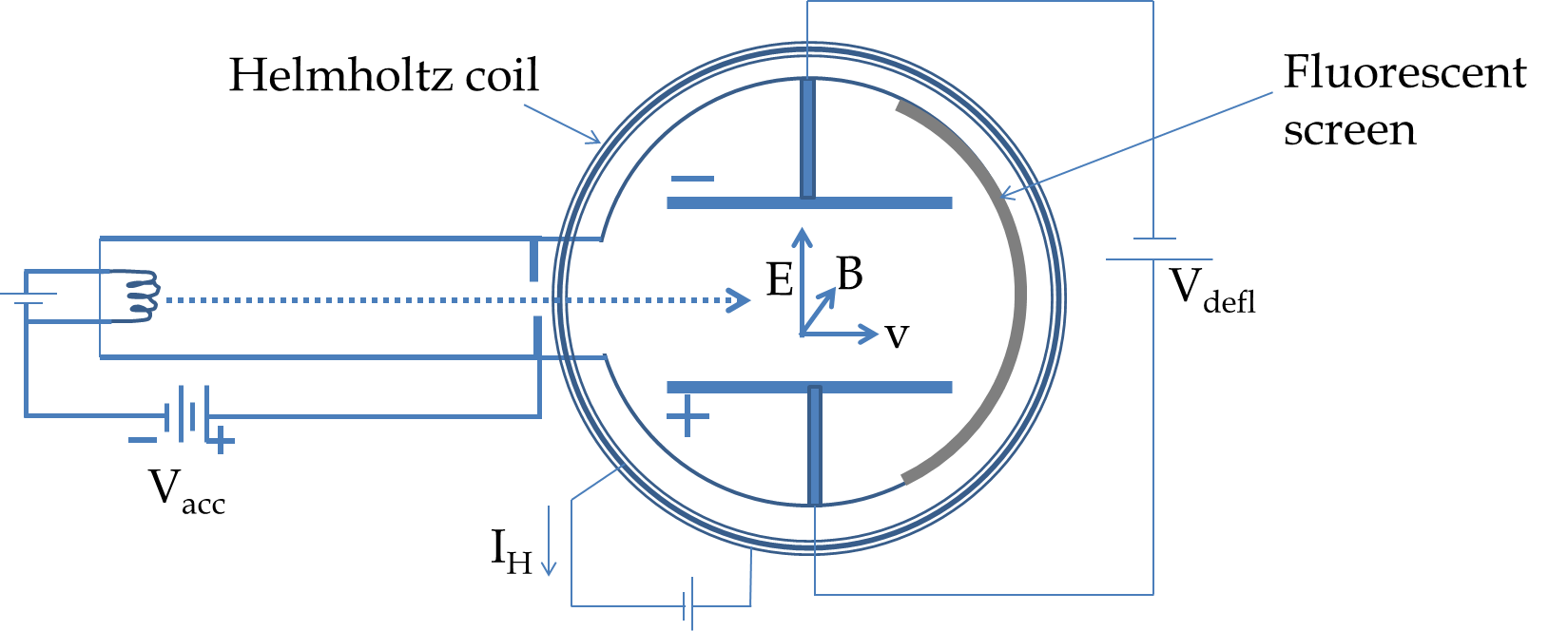## Section55.1The Discovery of Electron

Do atoms have parts? Prior to the work of J.J. Thomson and others 1880's and 1890's it was believed that atoms were the fundamental building blocks of nature. In 1838 Michael Faraday had observed that, when voltage was applied to two plates in a tube which was partially evacuated, a light arc appeared at the cathode (the negative plate) and almost reached the anode (the positive plate).

An improved version of Faraday's tube is a Crooke's tube shown in Figure 55.1.1. With an improved vacuum one can produce a stream of particles that starts from cathode and moves towards the anode by heating the cathode and applying a voltage between the cathode and the anode. If you cut a hole in the anode plate and place a fluorescent plate behind it, then you find that these particles pass through the anode and strike the fluorescent plate making the dye glow. These rays emitted by the cathode were known as cathode rays.Figure 55.1.1. A Crookes tube. The cathode rays travel from the cathode towards the anode and strike the right wall of the tube, which is coated with a fluorescing material. Credits: Wikicommons.

In 1890's J.J. Thomson conducted experiments on cathode rays to characterize the nature of particles that make up the rays. First, he demonstrated that the charge carried by cathode rays could not be separated from the cathode rays themselves. In another experiment he showed that the cathode rays passing through vacuum could be bent in an electric field just as electrically charged particles would.

From these two experiments, J.J. Thomson concluded, “I can see no escape from the conclusion that (cathode rays) are charges of negative electricity carried by particles of matter. ... What are these particles? Are they atoms, or molecules, or matter in a still finer state of subdivision?”

In an ingenious experiment, which is called the $e/m$ experiment now, he was able to measure the ratio of charge to mass $e/m$ for the particles of the cathode ray. He found that $e/m$ of these particles were more than a thousand times larger than that of a charged hydrogen atom, the lightest charged particle known at the time. Either the charged particles of the cathode ray had very small masses or were very highly charged.

In a modern version of the $e/m$ experiment shown in Figure 55.1.2 electrons from a heated metal filament are boiled off and accelerated to an evacuated chamber where a known electric field $\vec E$ bends them one way and a known magnetic field $\vec B$ bends them in the other way. By balancing the electric and magnetic forces we find their speed $v$ when the ray is not bent when passing through a region of crossed electric and magnetic fields of appropriate magnitudes. Let $q=-e$ be the charge on an electron, then balancing electric and magnetic forces gives us

\begin{equation} e E = e v B\ \ \Longrightarrow\ \ v = \frac{E}{B}.\label{eq-jjthomson-1}\tag{55.1.1} \end{equation}Figure 55.1.2. Schematic view of a modern version of the e/m experiment. The electrons from the heated cathode are accelerated by the accelerating voltage $V_{\textrm{acc}}\text{.}$ The cathode ray is deflected by electric field generated by $V_{\textrm{difl}}$ across plates and a uniform magnetic field generated by current through Helmholtz coil.

The speed of the changed particle can also be obtained from the radius of the circular orbit of the moving the particle in a uniform magnetic field by turning off the deflection voltage $V_{\textrm{defl}}$ in the apparatus shown in Figure 55.1.2. Assuming cathode rays start out nearly at rest before they are accelerated by the acceleration voltage $V_{\textrm{acc}}$ the speed with which they enter the evacuated tube will be based on the energy conservation in the acceleration process. Let $m$ be the mass of an electron. The conservation of energy of the electron gives

\begin{equation} \frac{1}{2} m v^2 = e V_{\textrm{acc}}, \label{eq-jjthomson-2}\tag{55.1.2} \end{equation}

which can be solved for $v$ to yield

\begin{equation} v = \sqrt{\frac{2q V_{\textrm{acc}}}{m}}. \label{eq-jjthomson-3}\tag{55.1.3} \end{equation}

From Eqs. (55.1.1) and (55.1.3) we obtain

\begin{equation} \frac{e}{m} = \frac{E^2}{2B^2V_{\textrm{acc}}}. \label{eq-jjthomson-4}\tag{55.1.4} \end{equation}

In the experimental arrangement given in Figure 55.1.2, the magnetic field is related to the number $N$ of windings in the Helmoholtz coil, the radius $R$ of the coils, and the current $I_H$ through the coils by

\begin{equation} B = \frac{8\:\mu_0}{5\sqrt{5}}\:\frac{N I_{H}}{R},\tag{55.1.5} \end{equation}

where

\begin{equation*} \mu_0 = 4\pi\times 10^{-7}\:\textrm{T.m/A}. \end{equation*}

The electric field $E$ from the deflection voltage $V_{\textrm{difl}}$ across the plates separated by a distance $d$ will be

\begin{equation} E = \frac{V_{\textrm{difl}}}{d}.\tag{55.1.6} \end{equation}

Thus, a measurement of $d\text{,}$ $V_{\textrm{difl}}\text{,}$ $I_H\text{,}$ $R\text{,}$ $N\text{,}$ and $V_{\textrm{acc}}$ yields the experimental value for $e/m$ from Eq. (55.1.4). The experiment is difficult to do precisely. Despite the impreciseness of the results, there was no doubt in Thomson's mind that cathode rays was made up of entirely new particles as he announced,

“We have in the cathode rays matter in a new state, a state in which the subdivision of matter is carried very much further than in the ordinary gaseous state: a state in which all matter... is of one and the same kind; this matter being the substance from which all the chemical elements are built up.”

In an e/m experiment accelerating voltage $V_{\textrm{acc}} = 3,000 \text{ V}\text{.}$ The accelerated electrons enter the evacuated chamber between the plates separated by a distance 5 cm. When a deviation voltage of $V_{\textrm{d}} = 100 \text{ V}$ is applied across the plates, the electric force acts on the moving electrons over a distance of 10 cm. (a) What is the transverse displacement of the electron? (b) What is the angle at which the electrons will emerge from the space between plates?

Hint

Ignore gravity and use constant acceleration.

(a) $\frac{1}{6}\:\textrm{cm}\text{,}$ (b) $1.9^{\circ}\text{.}$

Solution 1 (a)

We will refer to Figure 55.1.4 for calculations here. The acceleration part will be used to figure out the speed with which the electron will enter the deflection region. When the electron is moving between the plates, it has a constant force upward and gravity force downward. We will assume the electric force to be far greater than the force of gravity so that we can ignore the later. This gives a constant acceleration situation between the plates.

Using the consant acceleration equations will give us the vertical displacement when the horizontal displacement is $w = 10\text{ cm}\text{.}$ We will first work out the solution in symbolic form before we put in the numbers. The acceleration phase gives

\begin{equation*} \frac{1}{2} m v^2 = eV_{acc},\ \ \ v = \sqrt{\frac{2 eV_{acc}}{m}}. \end{equation*}

For calculations of motion in the space between plates let $x$-axis be pointed horizontally in the figure and $y$-axis pointed vertically up. Ignoring gravity, the acceleration of the electron when it is between plates will have only $y$-component,

\begin{equation*} a_y = \frac{eE}{m}, \end{equation*}

with

\begin{equation*} E = \frac{V_d}{d}. \end{equation*}

(a) To find the transverse displacement we apply constant velocity condition along the $x$-axis and constant acceleration condition along the $y$-axis yielding

\begin{equation*} w = v t,\ \ h = \frac{1}{2} a_y t^2. \end{equation*}

Eliminating $t$ gives

\begin{equation*} h = \frac{1}{2} a_y (w/v)^2. \end{equation*}

Now, plugging in $v$ and $a_y$ from above we get the vertical displacement to be

\begin{equation*} h = \frac{1}{2} \frac{eV_d}{m d} \:\frac{mw^2}{2 eV_{acc}} = \frac{1}{4} \frac{V_d w^2}{dV_{acc}}. \end{equation*}

The numerical values given here gives

\begin{equation*} h = \frac{1}{4} \frac{100\:\textrm{V}\times (10\:\textrm{cm})^2}{(5\:\textrm{cm})\times 3,000\:\textrm{V}} = \frac{1}{6}\:\textrm{cm}. \end{equation*}
Solution 2 (b)

(b) To find the direction in which the particle will be moving when it emerges from the space between the plates, we need the direciton of the velocity when the electron is there. We know the $x$-component to be $v\text{.}$

\begin{equation*} v_x = v = \sqrt{\frac{2 eV_{acc}}{m}}. \end{equation*}

The $y$-component will be

\begin{equation*} v_y = a_y t = \frac{eV_d}{m d}\: \frac{w}{v}. \end{equation*}

Therefore, the angle $\theta$ at which the electron will be headed will be

\begin{equation*} \tan\theta = \frac{v_y}{v_x} = \frac{eV_d}{m d}\: \frac{w}{v} \times \sqrt{\frac{m}{2 eV_{acc}}} = \frac{V_d w}{2 V_{acc} d}. \end{equation*}

For the numerical values given we get

\begin{equation*} \tan\theta = \frac{100\:\textrm{V}\times 10\:\textrm{cm} }{2\times 5\:\textrm{cm}\times 3,000\:\textrm{V}} = \frac{1}{30}. \end{equation*}

Therefore,

\begin{equation*} \theta \approx \frac{1}{30}\:\textrm{rad} = 1.9^{\circ}. \end{equation*}
TODO

An electron released at a cathode is accelerated by placing a voltage of 3,000 V across the anode and cathode. (a) What will be the speed of the electron when it strikes the anode? (b) Does it matter how far apart are the cathode and anode? Why or why not?

An electron is shot in a region of space with speed $1.5\times 10^5$ m/s where electric and magnetic field are uniform and perpendicular to each other. On the other side of the space the electron comes out with the same velocity as it enters the region. (a) If the electric field has the magnitude $3.0\times 10^4$ N/C, what will be the magnitude of the magnetic field? (b) Suppose the region of space is near Earth, the direction of the electric field is towards the East, and the electron enters the region from above. What is the direction of the magnetic field?

In a particular run of the Thomson's e/m experiment, an electron is accelerated by an acceleration voltage of 1,500 V. The electron's path is unchanged after passing through a region where there is a crossed electric and magnetic field which are perpendicular to each other and to the velocity of the electron. The electric field in the crossed field region is produced by placing 100 V across two plates that are separated by 1.5 cm distance. What must be the magnetic field in the crossed field region?

An electron with speed $v_0$ enters a space between two charges plates in which there is a uniform electric field of magnitude $E$ perpendicular to the velocity of the particle as shwon in Figure 55.1.9.

Let the width of the plates be $w$ and the separation between plates be sufficient that the electron emerges on the other side of where it enters. At what angle to the original direction would the electron come out?
An electron with speed $v_0$ enters a space between two charges plates in which there is a uniform electric field of magnitude $E$ perpendicular to the velocity of the particle. There is also a uniform magnetic field of magnitude $B$ perpendicular to the electric field as shown in Figure 55.1.11.
Let the width of the plates be $w$ and the separation between plates be sufficient that the electron emerges on the other side of where it enters. At what angle to the original direction would the electron come out?